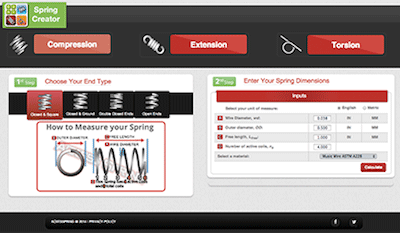<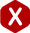# Coil Springs Mean Diameter

#### Definition: Coil spring specification that measures the diameter in between the spring’s outer and inner diameters.

Mean diameter is used to calculate several formulas which are necessary to your design such as index and wire length for manufacturing reasons, or spring rate to know your spring’s force and working loads.

Coil spring mean diameter can be measured many ways. It is like your spring’s outer and inner diameters except, instead of measuring from the outside of the coil or the inside of the coil, you will measure from in between these spring dimensions; from the center of the coil’s wire. The formula and diagram to calculate coil spring mean diameter are the following.

To calculate mean diameter you must simply either subtract one wire diameter from the outer diameter or add one wire diameter to the inner diameter.

#### Coil Spring Mean Diameter Formula

Mean Diameter = Outer Diameter – Wire Diameter
Mean Dianmeter = Inner DIameter + Wire Diameter
##### Example

You have a spring with a wire diameter of 0.035 inches, an outer diameter of 0.500 inches, and an inner diameter of 0.430 inches.

MD = OD – WD
MD = 0.500 – 0.035
MD = 0.465

MD = ID + WD
MD = 0.430 + 0.035
MD = 0.465

#### Formula Diagram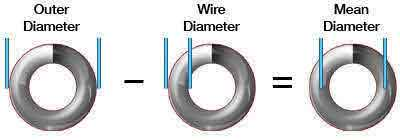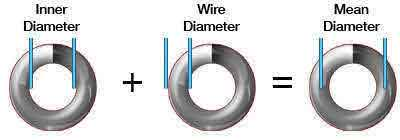Below, you will find the explanations of the formulas in which the coil spring mean diameter dimension is needed in order to calculate an important specification to your coil spring design.

#### Coil Spring Index Formula

To calculate spring index you must divide the mean diameter by the wire diameter as the formula shows below.

I = MD ÷ WD
##### Example

You have two springs. Spring A has a mean diameter of 2.750" inches and a wire diameter of 0.250 inches. Spring B has a mean diameter of 2.500 inches and a wire diameter of 0.500 inches.

Spring A

I = MD ÷ WD
I = 2.75 ÷ 0.25
I = 11 to 1

Spring B

I = MD ÷ WD
I = 2.5 ÷ 0.5 I = 5 to 1

#### Formula Diagram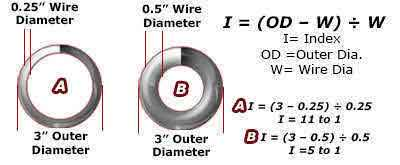#### Coil Spring Wire Length

To calculate your coil spring’s wire length using coil spring mean diameter, you must multiply the mean diameter by pi and multiply the product by the total number of coils.

Wire Length = Mean Diameter x Pi x Total Coils

##### Example

You have a mean diameter of 0.465 inches and 10 total coils.

WL = 3.14 MD(TC)
I = 3.14 x 0.465 x 10
I = 14.601 inches

#### Get our New Free Spring Creator App for Android and iOS Devices#### Coil Spring Rate Formula

To calculate your coil spring’s rate there are several factors to consider besides your coil spring mean diameter. The formula along with symbol nomenclature is shown below.

D = D outer – d
G = E ÷ 2(1 + V)
k = Gd^4 ÷ (8D^3 x na)

##### Nomenclature
• d = Wire Diameter
• D outer = Outer Diameter
• D = Mean Diameter
• E = Young's Modulus of Material
• G = Shear Modulus of Material
• k = Spring Rate
• na = Active Coils
• V = Poison's Ration of Material

#### Formula Diagram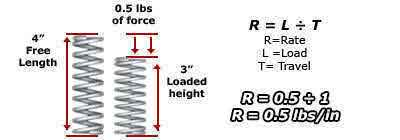#### Spring Rate Calculator